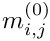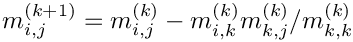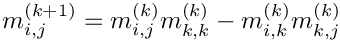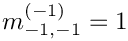GiNaC 1.8.7
GiNaC::solve_algo Class Reference

Switch to control algorithm for linear system solving. More...

`#include <flags.h>`

## Public Types

enum  {
automatic , gauss , divfree , bareiss ,
markowitz
}

## Detailed Description

Switch to control algorithm for linear system solving.

Definition at line 141 of file flags.h.

## ◆ anonymous enum

 anonymous enum
Enumerator
automatic

Let the system choose.

A heuristics is applied for automatic determination of a suitable algorithm.

gauss

Gauss elimination.

Ifare the entries of the original matrix, then the matrix is transformed into triangular form by applying the rulesThis algorithm is well-suited for numerical matrices but generally suffers from the expensive division (and computation of GCDs) at each step.

divfree

Division-free elimination.

This is a modification of Gauss elimination where the division by the pivot element is not carried out. Ifare the entries of the original matrix, then the matrix is transformed into triangular form by applying the rulesThis algorithm is only there for the purpose of cross-checks. It suffers from exponential intermediate expression swell. Use it only for small systems.

bareiss

Bareiss fraction-free elimination.

This is a modification of Gauss elimination where the division by the pivot element is delayed until it can be carried out without computing GCDs. Ifare the entries of the original matrix, then the matrix is transformed into triangular form by applying the rules(We have setin order to avoid a case distinction in above formula.) It can be shown that nothing more than polynomial long division is needed for carrying out the division. This is generally the fastest algorithm for solving linear systems. In contrast to division-free elimination it only has a linear expression swell. For two-dimensional systems, the two algorithms are equivalent, however.

markowitz

Markowitz-ordered Gaussian elimination.

Same as the usual Gaussian elimination, but with additional effort spent on selecting pivots that minimize fill-in. Faster than the methods above for large sparse matrices (particularly with symbolic coefficients), otherwise slightly slower than Gaussian elimination.

Definition at line 143 of file flags.h.

The documentation for this class was generated from the following file:

This page is part of the GiNaC developer's reference. It was generated automatically by doxygen. For an introduction, see the tutorial.